# How to divide a range of cells by a number in Excel?

It is possible that we will have scenarios where we need to make rapid changes to a range of cells in Excel. For example, there are multiple tables in some of the worksheets that have values that are in six digit numbers and you want to decrease them to, by dividing with some number, in these cases you have to perform the division operation taking the cell ranges rather than dividing that number to each cell separately.

Let’s understand stepwise with an example.

## Using Paste Special

You can use the Paste Special feature to divide a range of cells by a number.

Step 1

In our example, we have the MRP of some item and we need to calculate the discount price of each item. Let’s consider each item having a discount of 20%, which means we have to divide the MRP of each item with 5. See the below given image for reference.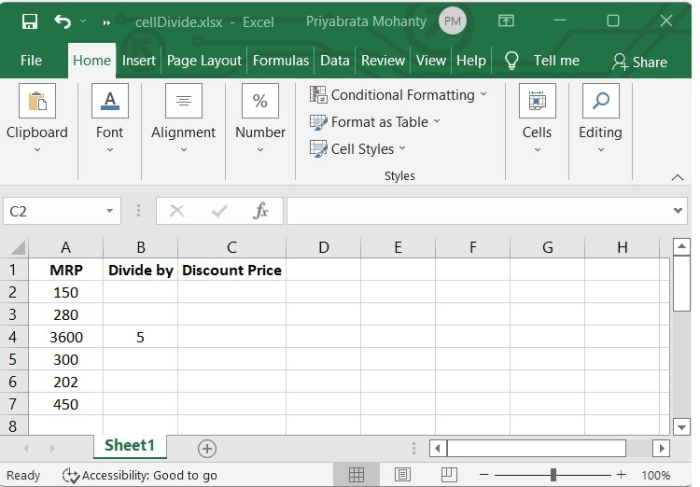Step 2

In our example, we have copied all the MRP values to Discount Price column so that it will be easy to use the paste special option and also, we can differentiate between MRP and Discount Price.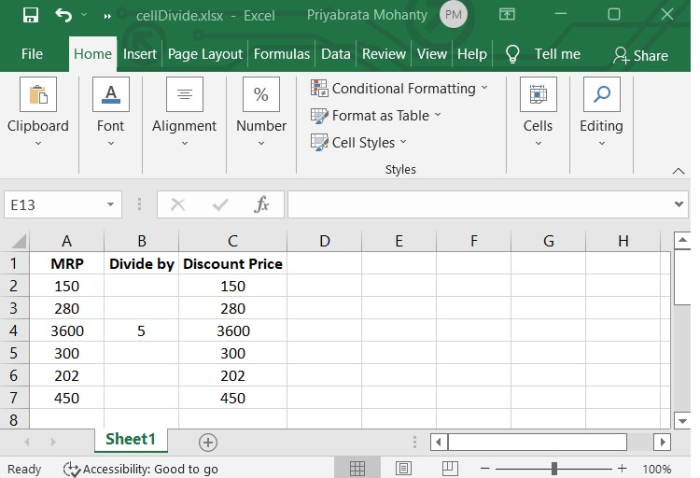Step 3

Now select 5 and copy it. Then select the numbers from Discount Price column and right click there. You will see a Paste Special option, click there.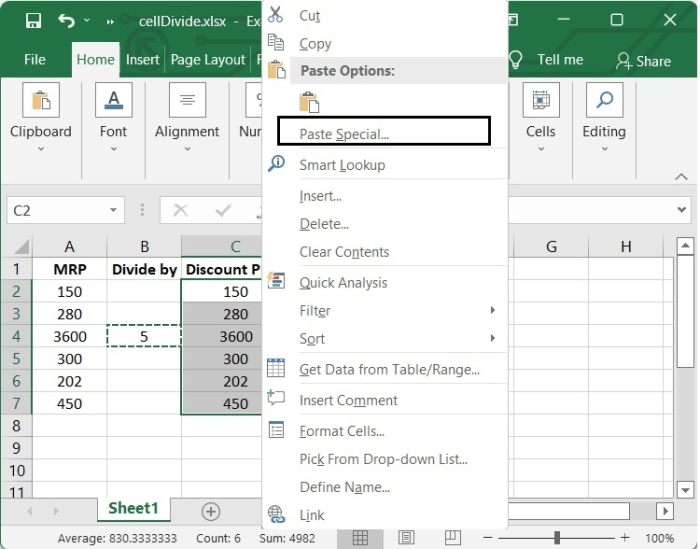Step 4

After Clicking on Paste Special option, one popup paste special menu will be opened, and then select “all” option, then Click on “divide” option and click OK. See the below given image.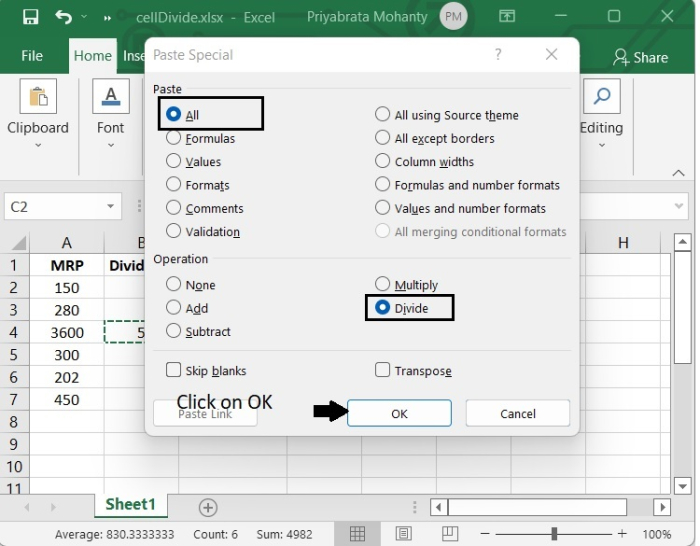After clicking OK, we will see the result in Discount price column.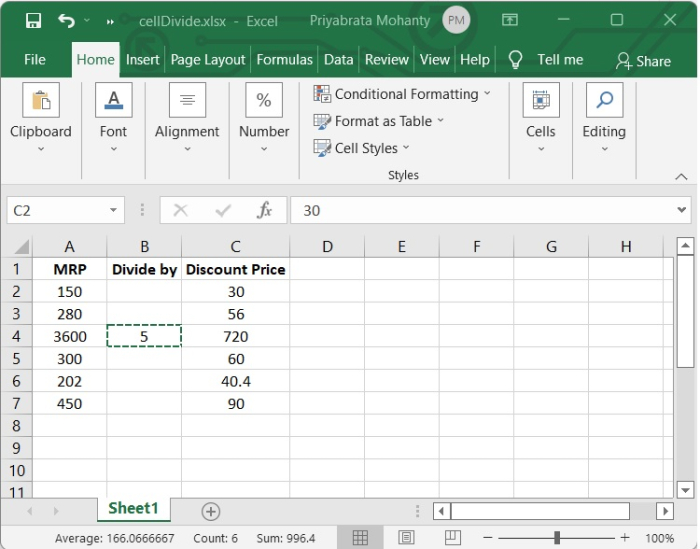## Using Formula

We also have the option of making use of Excel formulas in order to generate a new range. This has the advantage of maintaining the values from the beginning. In the event that it becomes necessary, we also have the ability to dynamically adjust the divisor.

See the below given steps to get a clear idea.

Step 1

In the formula bar, type the formula "=A2/$B$$B$4", as shown in the following image −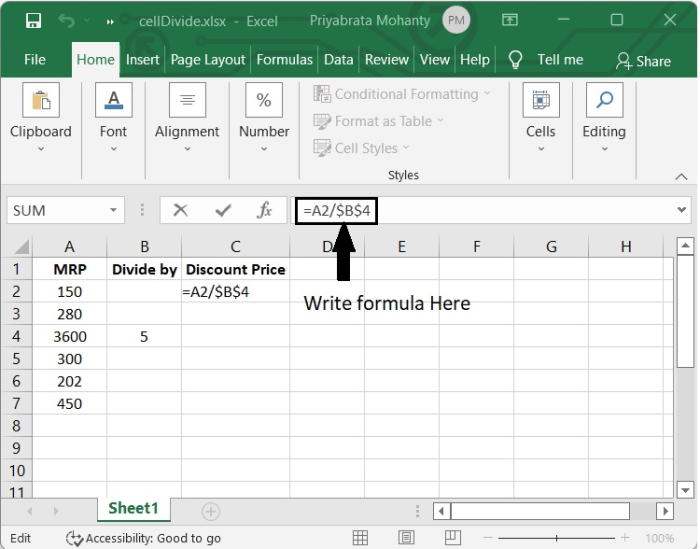Step 2

After pressing Enter, we can see the result in that cell.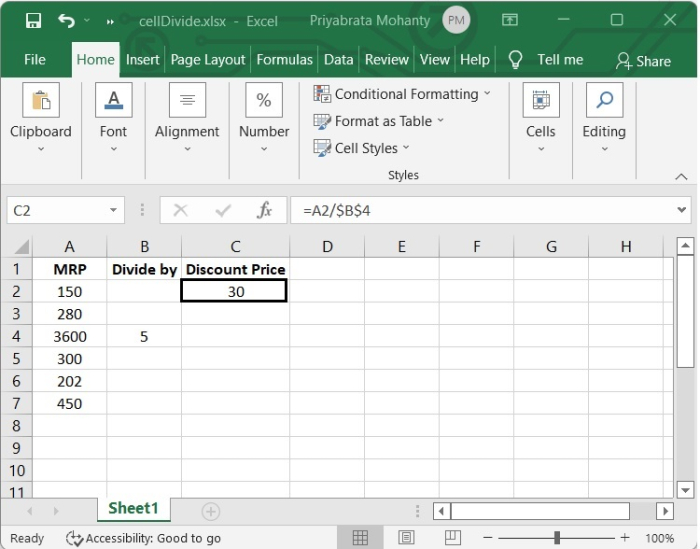Step 3

Click on the lower-right corner and drag down to the last required cell.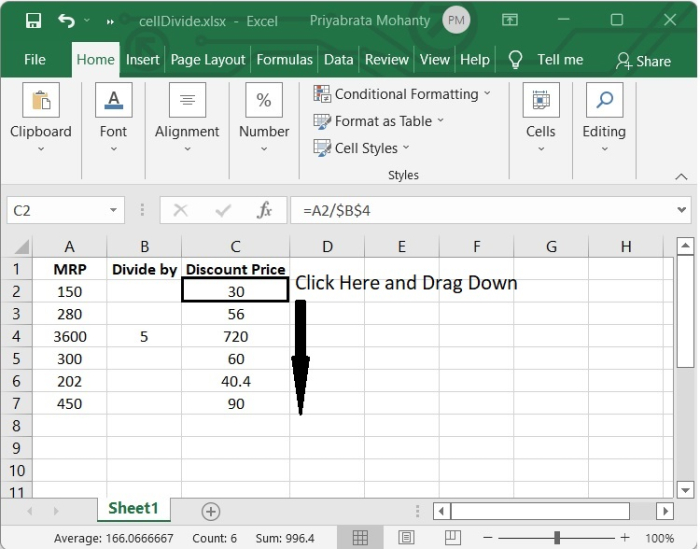## Conclusion

In this tutorial, we explained in detail how you can divide a range of cells in Excel by a number.